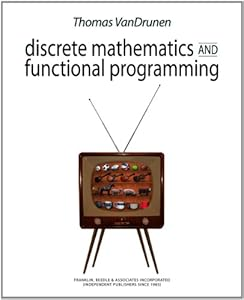# Discrete Mathematics and Functional Programming9781590282601

Discrete Mathematics and Functional Programming
by: Thomas VanDrunen
ISBN-10 书号： 1590282604
ISBN-13 书号： 9781590282601
Release Finelybook 出版日期： 2012-10-16
pages 页数： 688
This book provides a distinct way to teach discrete mathematics. Since discrete mathematics is crucial for rigorous study in computer science,many texts include applications of mathematical topics to computer science or have selected topics of particular interest to computer science. This text fully integrates discrete mathematics with programming and other foundational ideas in computer science. In fact,this text serves not only the purpose of teaching discrete math. It is also an introduction to programming,although a non-traditional one. Functional programming is a paradigm in which the primary language construct is the function and function here is essentially the same as what it is in mathematics. In the functional paradigm we conceive the program as a collection of composed functions,as opposed to a sequence of instructions (in the imperative paradigm) or a set of interacting objects (in the object-oriented paradigm). Dominant computer science curricula emphasize object-oriented and imperative programming,but competence in all paradigms is important for serious programmers and functional programming in particular may be appropriate for many casual programmers,too. For our purposes,the concepts underlying functional programming are especially grounded in those of discrete mathematics. Discrete mathematics and functional programming are equal partners in this endeavor,with the programming topics giving concrete applications and illustrations of the mathematical topics,and the mathematics providing the scaolding for explaining the programming concepts. The two work together in mutual illumination.

## 下载地址：

Discrete Mathematics and Functional Programming 9781590282601.pdf

### 评论 抢沙发

#### 支付宝扫一扫打赏#### 微信扫一扫打赏